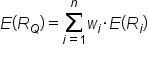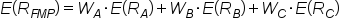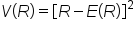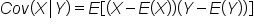+

# Implications Across Portfolios

Author: Sophia Tutorial
##### Description:

Understand the various measures of portfolio risk, including variance, covariance, and a beta coefficient.

(more)### Developing Effective Teams

*No strings attached. This college course is 100% free and is worth 1 semester credit.

37 Sophia partners guarantee credit transfer.

299 Institutions have accepted or given pre-approval for credit transfer.

* The American Council on Education's College Credit Recommendation Service (ACE Credit®) has evaluated and recommended college credit for 33 of Sophia’s online courses. Many different colleges and universities consider ACE CREDIT recommendations in determining the applicability to their course and degree programs.

Tutorial

what's covered
In this lesson, you will learn about the various measures of portfolio risk, including variance, covariance, and a beta coefficient. Specifically, this lesson will cover:
1. Calculating Expected Portfolio Returns
2. Calculating Portfolio Risk
3. Beta Coefficient for Portfolios

## 1. Calculating Expected Portfolio Returns

The formula for calculating an expected portfolio return uses the expected return formula that has been previously discussed:

formula

Expected Portfolio ReturnThis formula can be used to calculate the expected return on a portfolio, Q, of n assets, where wi is the weight and E(Ri) is the expected return of an asset.

Let's look at an example:

### IN CONTEXT

Suppose a farmer's market portfolio consists of three assets:
• Apples
• Bananas
• Cherries
We decided to invest in all three, because the previous chapters on diversification had a profound impact on our investment strategy, and we now understand that diversifiable risk doesn’t pay a risk premium, so we try to eliminate it.

Fruit Investment Weight Expected Return
Apples \$50,000 0.50 12%
Bananas \$25,000 0.25 6%
Cherries \$25,000 0.25 10%

How would you calculate the expected return on this portfolio?

The return of our fruit portfolio could be modeled as a sum of the weighted average of each fruit’s expected return. In math, that means:Calculate this figure on your own and you should have gotten an expected return on the farmer's market portfolio of 0.1, or 10%:Remember that we are making the assumption that we can accurately measure these outcomes based on what we have seen in the past.

EXAMPLE

If you were playing roulette at a casino, you may not know if red or black (or green) is coming on the next spin, but you could reasonably expect that if you bet on black 4,000 times in a row, you’re likely to get paid on about 1,900 of those spins.

If you go to Wikipedia, you can review a wide variety of challenges to this model that have very valid points. Remember, the market is random: it is not a roulette wheel, but that might be the best thing we have to compare it to.

## 2. Calculating Portfolio Risk

In order to bring down levels of portfolio risk, investors can engage in a variety of investments whose correlation is not perfectly aligned. This has the effect of reducing the phenomenon of individual asset risk by investing in a portfolio of diversified assets with varying correlations. This provides an opportunity for the same level of return but with a lower risk premium.

### IN CONTEXT

Three assets – apples, bananas, and cherries – can be thought of as a bowl of fruit. The index is a fruit basket. A full fruit basket probably has 10 or 15 different fruits, but my bowl will be efficient as much as its statistical parameters (risk and return) mimic those of the whole basket.

How does my bowl of fruit compare to the whole basket and how does that compare to other bowls out there?

To calculate the risk in my bowl, we need a little more background information on fruit markets. First, we are going to need the variance for each fruit. Remember that the standard deviation answers the question of how far do I expect one individual outcome to deviate from the overall mean, and the variance is that number squared. Mathematically, the formula is:

 formula VarianceIt is the expected value of the difference between the individual return in a given day (R) and the average outcome average return over a year (E(R)).

In order to calculate the variance of a portfolio of three assets, we need to know that figure for apples, bananas, and cherries, and we also need to know the covariance of each. Covariances can be thought of as correlations. If every time bananas have a bad day, so do apples, their covariance will be large. If bananas do great half of the time when cherries do badly and bananas do terribly the other half, their covariance is zero. If there is zero correlation among all three fruits, we have cut our risk in thirds by owning all three, but if they are perfectly correlated, we haven’t diversified away any of our risk.

In reality, they are probably positively correlated, since they are all fruits, but not at all perfectly. Apples and bananas grow in different climates so their performance may be a result of weather patterns in either region. Apples may be a substitute for cherries when cherries are expensive. The overall risk of the portfolio would take into account three individual variances and three covariances (apples-bananas, apples-cherries, and bananas-cherries) and it would reduce the overall portfolio to the degree that they are uncorrelated.

The formula to compute the covariance between returns on X and Y:

 formula CovarianceSo, now that we have figures to help us measure the risk and reward of our individual fruit bowl, we can go look at historical figures to determine the expected returns and the risk of the index that comprises the entire fruit basket industry. If our portfolio of investments has diversified away as much risk as is possible given the costs of diversifying, our portfolio will be attractive to investors. If our bowl does not diversify away enough risk, it will not lie on the security market line (SML) for those who we are trying to recruit into buying our portfolio.

big idea
If an institutional investor, such as a city pension fund, looked at two portfolios with identical returns and different risks, they would choose the portfolio that minimized its risk. Thus, the only portfolios that are efficient investments are those that effectively diversify the underlying risk away and price their investment efficiently.

term to know

Covariance
A measure of how much two random variables change together.

## 3. Beta Coefficient for Portfolios

In this section, we will discuss the idea of calculating a beta coefficient to help investors measure the risk-reward trade-off for a blended pool of investments.

In this case, it is important to remember that a portfolio may represent the seller side of the market and the buyer can be thought of an institutional investor or a mutual fund. A portfolio’s beta is the volatility correlated to an underlying index. If we think of the S&P 500 as the index, a portfolio that fluctuates identically to the market has a beta of 1.

What would the following portfolios have for beta values?

 S&P 500 Portfolio A Portfolio B +2% +6% -1% -1% -3% +0.5% +3% -9% -1.5%

Beta is a normalized variable, which means that it is a ratio of two variances, so you have to compare the volatility of returns to the benchmark volatility.

• Portfolio A has a direct relationship with the S&P 500 – it is scaled by it three times each day. When the market is up 2%, it is up 6%. Thus, the portfolio would have a beta value of 3.
• Portfolio B is a different situation; it is also directly proportional, but in the negative direction. Every time the market is up 1%, the portfolio is down half a percent. The beta for this portfolio, when compared with the S&P 500 benchmark, would be -0.5.
A beta of zero in this situation doesn’t necessarily mean a risk-free asset, it simply means that it is not correlated with the benchmark.

summary
In this lesson, you learned how to calculate expected portfolio returns by adding together the weighted average of each asset’s expected return. Investors can also calculate portfolio risk and pursue investment strategies to reduce portfolio risk by determining the variance and covariance of the assets within the portfolio and ensuring it contains diversified assets with varying correlations. The beta coefficient for portfolios provides investors with information regarding the correlation of a portfolio with a benchmark like the S&P 500.

Best of luck in your learning!

Source: THIS CONTENT HAS BEEN ADAPTED FROM LUMEN LEARNING'S “Implications Across Portfolios” TUTORIAL.

Terms to Know
Covariance

A measure of how much two random variables change together.

Formulas to Know
CovarianceExpected Portfolio ReturnVarianceRating